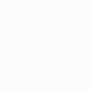# two YAxes with XAxis as DateTime type, the linear series won't show

0
0

I have two questions:

1. If I only use one YAxies, everything works fine. If I use two YAxes, one aligns on the left, on aligns on the right. The
simple liner series won’t show. Code is below:

XAML:

``````        <!--  Create an X Axis with Growby  -->
<s:SciChartSurface.XAxis>
<s:DateTimeAxis TextFormatting="dd MMM" SubDayTextFormatting="HH:mm:ss" CursorTextFormatting="dd MMM HH:mm:ss"/>
</s:SciChartSurface.XAxis>

<!--  Create a Y Axis with Growby. Optional bands give a cool look and feel for minimal performance impact
<s:SciChartSurface.YAxes>
<s:NumericAxis AxisAlignment="Left"/>
<s:NumericAxis AxisAlignment="Right"/>
</s:SciChartSurface.YAxes> -->

<s:SciChartSurface.YAxis>
<s:NumericAxis AxisAlignment="Right"/>
</s:SciChartSurface.YAxis>
<s:SciChartSurface.Annotations>
<s:TextAnnotation FontSize="12" Text="Draw boxes" X1="0" Y1="10"/>
<s:BoxAnnotation Background="#55279B27" BorderBrush="#279B27" BorderThickness="1" CornerRadius="3" X1="3.5" X2="5" Y1="4" Y2="5"/>

<s:BoxAnnotation Background="#55FF1919" BorderBrush="#FF1919" BorderThickness="1" CornerRadius="3" X1="4" X2="5.5" Y1="4.5" Y2="5.5"/>

<s:BoxAnnotation Background="#551964FF" BorderBrush="#1964FF" BorderThickness="1" CornerRadius="3" X1="4.5" X2="6" Y1="5" Y2="6"/>
</s:SciChartSurface.Annotations>
</s:SciChartSurface>
</Grid>
``````

Backend Code:

private void LineChartExampleView_OnLoaded(object sender, RoutedEventArgs e) {
double[] yData = new double;
DateTime[] xData = new DateTime;
// Create a DataSeries of type X=double, Y=double
var dataSeries = new XyDataSeries<DateTime, double>();

``````        lineRenderSeries.DataSeries = dataSeries;

for (int i = 0; i < 100; i++) {
yData[i] = Convert.ToDouble(i);
dataSeries.Append(xData[i], yData[i]);
}

sciChart.ZoomExtents();
}
``````

This is the work version. If I change the xaml YAxes to two as in the comments, the liner series won’t show.

1. How to add a horizontal stack bar with different color when the XAxies is DataTime type? I tried to use annotation,
but don’t know how. Please check attachment “HorizontalBar” picture. Also the two set of YAxes should have different
scales.

Thanks!

• You must to post comments
0
0

Hi there,

If you look at the output window you probably see an exception which says that you have two YAxis with the same AxisId (or similar). As a hint, if SciChart is ever blank you can get it to tell you why the chart is blank.

All you need to do is assign Axis Id to the secondary axis and then assign YAxisId to the RenderableSeries (if you wish to place it on the secondary YAxis).

Best regards,
Andrew

•I made changes according to your answer, right now the liner series shows, but the YAXes scales aren’t right, I want Left YAxies scales at 10, right YAxies scales at 0.1, Please see revised code. Plus my second question hasn’t answered yet: How to draw a horizontal stack bar with different color as showed in attachment picture. XAML: Backend C#: private void LineChartExampleView_OnLoaded(object sender, RoutedEventArgs e) { double[] yData = new double; DateTime[] xData = new DateTime; // Create a DataSeries of type X=double, Y=double var dataSeries = new XyDataSeries(); lineRenderSeries.DataSeries = dataSeries; for (int i = 0; i < 100; i++) { yData[i] = Convert.ToDouble(i); xData[i] = DateTime.Now.AddMinutes(i); dataSeries.Append(xData[i], yData[i]); } sciChart.ZoomExtents(); }
•Hi there, you’re not really helping us with these blocks of code!! Can you please take a look at https://www.scichart.com/how-to-post-code-samples-in-support-tickets/ and ensure code formats correctly!! .. To set Axis scaling you need to set Axis.VisibleRange. You can find documentation here https://www.scichart.com/documentation/v4.x/webframe.html#Axis%20Ranging%20-%20AutoRange%20and%20VisibleRange.html Best regards, Andrew
• You must to post comments
Showing 1 result